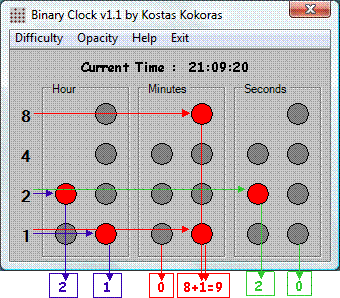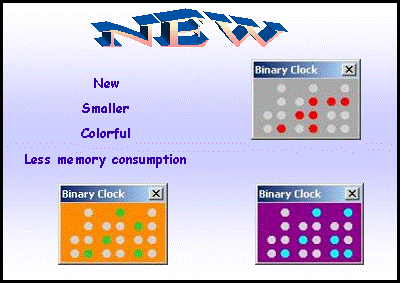· What is it?            It is just another way to display the time, using a more complicated method. It uses the famous BCD method (Binary Coded to Decimal) by converting each decimal digit of time to binary and displaying it graphically. That’s way it’s called Binary Clock.   · How it works?            Let’s take as example the image above. Each column represents a digit. Two columns (digits) for Hour, as for Minutes and seconds. The four numbers on the left side (1 2 4 8) represent the value of each bit. Every bit is red means that is 1 and it takes the value that represents. If more than one bit is red then you add the values and you get the final decimal digit.   · Example.            Lets take as example the minutes. The minutes has two digits. At the first digit no bit is red so it takes no value and it is 0 (zero). At the second digit two bits is red and they take the values 8 and 1. If you add them you get the number 9. So the minutes are 09.